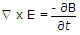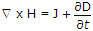# Electronics and Communication Engineering - Exam Questions Papers

16.

For pole-zero plot shown, the system is __________ .A. minimum phase B. maximum phase C. mixed phase D. none of these

Answer: Option A

Explanation:

No answer description available for this question. Let us discuss.

17.

Which of the following equations results from the circuital form of Ampere's law?

 A.B. ∇ . B = 0 C. ∇ . B = r D.Answer: Option D

Explanation:

No answer description available for this question. Let us discuss.

18.

Which one of the following is not LED material?

 A. GaAs B. GaP C. SiC D. SiO2

Answer: Option D

Explanation:

No answer description available for this question. Let us discuss.

19.

Consider the following statements:

1. A flip-flop is used to store 1-bit of information.
2. Race-around condition occurs in a J-K flip-flop when both the inputs are 1.
3. Master-slave configuration is used in flip-flops to store 2-bits of information
4. A transparent latch consists of a D-type flip-flop.
Which of the above statements is/are correct?

 A. 1 only B. 1, 3 and 4 C. 1, 2 and 4 D. 2 and 3 only

Answer: Option C

Explanation:

No answer description available for this question. Let us discuss.

20.

A balanced modulator is used in the generation of which of the following?

 A. DSB-SC signal B. FM signal C. PM signal D. PAM signal

Answer: Option A

Explanation:

No answer description available for this question. Let us discuss.

#### Current Affairs 2021

Interview Questions and Answers